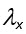## DETAILED DEFINITION OF RISK INDICES USED IN GIS MAPPING

Establishment risk index

The establishment risk index (ERI) is defined as the number of time intervals with a net reproduction rate, Ro, above 1 (Ii=1) divided by the total number of time intervals within a year (Ii). By default, the maps, as presented in the Atlas, are generated by using a 1-month time scale; however, the calculation can also be based on other time scales (e.g., 1-day intervals). The formula for using monthly intervals is as follows:in which Ii is the interval of the month i (with i = 1, 2, 3, …, 12). Its value is 1 if the population is expected to increase within this interval (Ii = 1 if Ro ≥ 1), and the value is 0 if the population is expected to decrease (Ii = 0 if Ro < 1) according to the established temperature-driven phenology model, and the total number of intervals, II, is 12.

Generation index

The generation index (GI) is computed by averaging the sum of estimated generation lengths, Ti, calculated for each Julian day, x , as shown in the following expression:where 365 is the number of days per year and Ti is the predicted average generation lengths in days during the month, i (i = 1, 2, 3, …, 12).

Activity index

The activity index (AI) is computed by employing the following equation:whereis the finite rate of increase at Julian day, x (x = 1, 2, 3, …, 365).

whereis the finite rate of increase per day during month i (i = 1, 2, 3, …, 12).